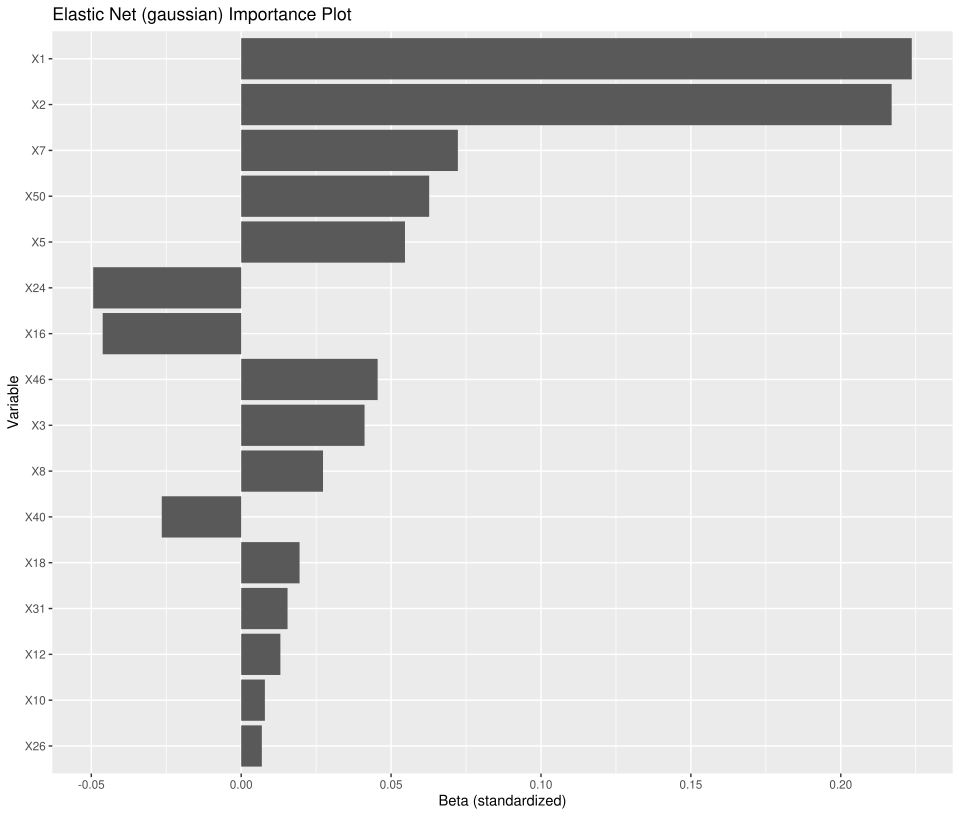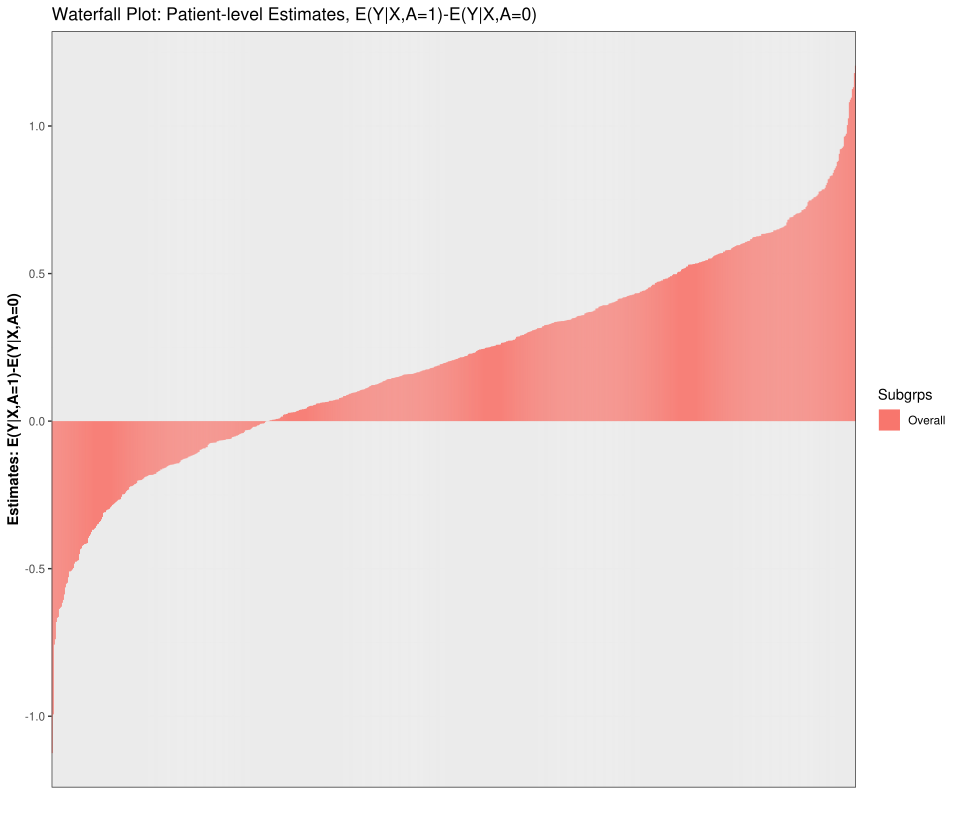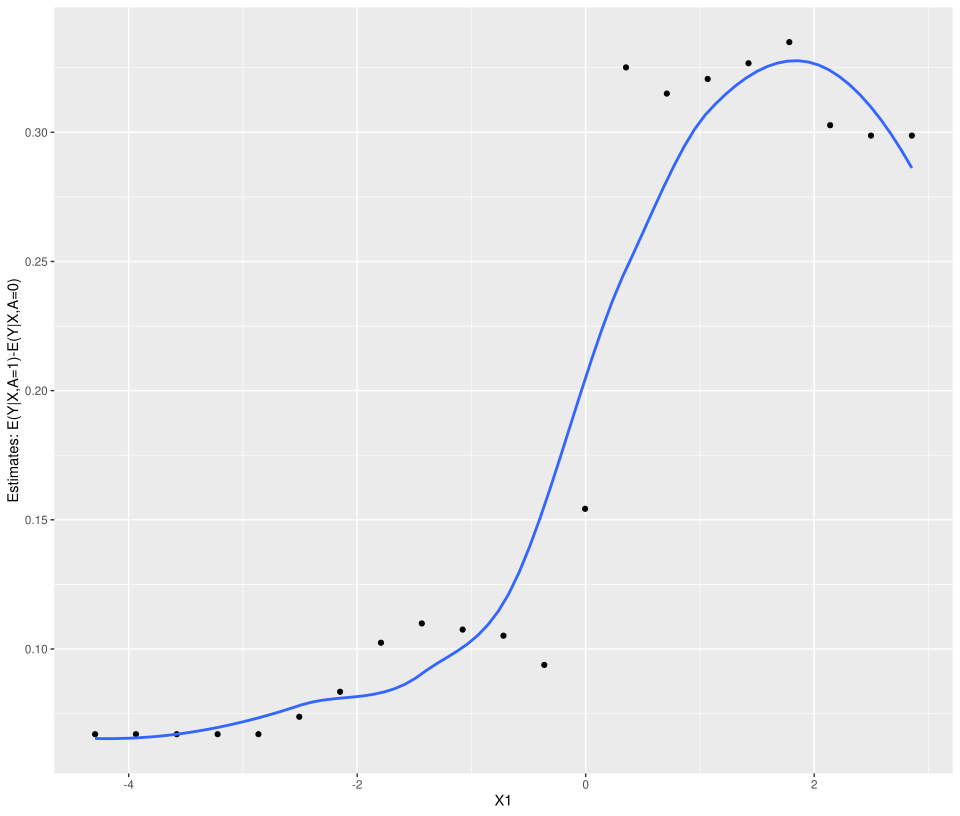# Introduction

Welcome to the StratifiedMedicine R package. The overall goal of this package is to develop analytic and visualization tools to aid in stratified and personalized medicine. Stratified medicine aims to find subsets or subgroups of patients with similar treatment effects, for example responders vs non-responders, while personalized medicine aims to understand treatment effects at the individual level (does a specific individual respond to treatment A?).

Currently, the main tools in this package area: (1) Filter Models (identify important variables and reduce input covariate space), (2) Patient-Level Estimate Models (using regression models, estimate counterfactual quantities, such as the conditional average treatment effect or CATE), (3) Subgroup Models (identify groups of patients using tree-based approaches), and (4) Parameter Estimation (across the identified subgroups), and (5) PRISM (Patient Response Identifiers for Stratified Medicine; combines tools 1-4). Development of this package is ongoing.

As a running example, consider a continuous outcome (ex: % change in tumor size) with a binary treatment (study drug vs standard of care). The estimand of interest is the average treatment effect, $$\theta_0 = E(Y|A=1)-E(Y|A=0)$$. First, we simulate continuous data where roughly 30% of the patients receive no treatment-benefit for using $$A=1$$ vs $$A=0$$. Responders vs non-responders are defined by the continuous predictive covariates $$X_1$$ and $$X_2$$ for a total of four subgroups. Subgroup treatment effects are: $$\theta_{1} = 0$$ ($$X_1 \leq 0, X_2 \leq 0$$), $$\theta_{2} = 0.25 (X_1 > 0, X_2 \leq 0)$$, $$\theta_{3} = 0.45 (X_1 \leq 0, X2 > 0$$), $$\theta_{4} = 0.65 (X_1>0, X_2>0)$$.

library(ggplot2)
library(dplyr)
library(partykit)
library(StratifiedMedicine)
library(survival)
dat_ctns = generate_subgrp_data(family="gaussian")
Y = dat_ctns$Y X = dat_ctns$X # 50 covariates, 46 are noise variables, X1 and X2 are truly predictive
A = dat_ctns$A # binary treatment, 1:1 randomized length(Y) #>  800 table(A) #> A #> 0 1 #> 409 391 dim(X) #>  800 50 # Filter Models The aim of filter models is to potentially reduce the covariate space such that subsequent analyses focus on the “important” variables. For example, we may want to identify variables that are prognostic and/or predictive of the outcome across treatment levels. Filter models can be run using the “filter_train” function. The default is search for prognostic variables using elastic net (Y~ENET(X); Hou and Hastie 2005). Random forest based importance (filter=“ranger”) is also available. See below for an example. Note that the object “filter.vars” contains the variables that pass the filter, while “plot_importance” shows us the relative importance of the input variables. For glmnet, this corresponds to the standardized regression coefficients (variables with coefficients=0 are not shown). res_f <- filter_train(Y, A, X, filter="glmnet") res_f$filter.vars
#>   "X1"  "X2"  "X3"  "X5"  "X7"  "X8"  "X10" "X12" "X16" "X18" "X24" "X26"
#>  "X31" "X40" "X46" "X50"
plot_importance(res_f)An alternative approach is to search for variables that are potentially prognostic and/or predictive by forcing variable by treatment interactions, or Y~ENET(X,XA). Variables with estimated coefficients of 0 in both the main effects (X) and interaction effects (XA) are filtered. This can be implemented by tweaking the hyper-parameters:

Here, note that both the main effects of X1 and X2, along with the interaction effects (labeled X1_trtA and X2_trtA), have relatively large estimated coefficients.

# Patient-level Estimate (PLE) Models

The aim of PLE models is to estimate counterfactual quantities, for example the CATE. This is implemented through the “ple_train” function. The “ple_train” follows the framework of Kunzel et al 2019, which utilizes base learners and meta learners to obtain estimates of interest. For family=“gaussian”, “binomial”, this output estimates of and treatment differences. For family=“survival”, either logHR or restricted mean survival time (RMST) estimates are obtained. Current base-leaner options include “linear” (lm/glm/or cox), “ranger” (random forest through ranger R package), “glmnet” (elastic net), and “bart” (Bayesian Additive Regression Trees through BART R package). Meta-learners include the “T-Leaner” (treatment specific models), “S-learner” (single regression model), and “X-learner” (2-stage approach, see Kunzel et al 2019). See below for an example. Note that the object “mu_train” contains the training set patient-level estimates (outcome-based and propensity scores), “plot_ple” shows a waterfall plot of the estimated CATEs, and “plot_dependence” shows the partial dependence plot for variable “X1” with respect to the estimated CATE.

res_p1 <- ple_train(Y, A, X, ple="ranger", meta="T-learner")
summary(res_p1\$mu_train)
#>       mu_0             mu_1           diff_1_0             pi_0
#>  Min.   :0.6493   Min.   :0.5308   Min.   :-1.12502   Min.   :0.5112
#>  1st Qu.:1.4166   1st Qu.:1.5707   1st Qu.:-0.02003   1st Qu.:0.5112
#>  Median :1.6217   Median :1.8048   Median : 0.20715   Median :0.5112
#>  Mean   :1.6340   Mean   :1.8458   Mean   : 0.21181   Mean   :0.5112
#>  3rd Qu.:1.8422   3rd Qu.:2.1153   3rd Qu.: 0.46391   3rd Qu.:0.5112
#>  Max.   :2.6318   Max.   :3.0649   Max.   : 1.20451   Max.   :0.5112
#>       pi_1
#>  Min.   :0.4888
#>  1st Qu.:0.4888
#>  Median :0.4888
#>  Mean   :0.4888
#>  3rd Qu.:0.4888
#>  Max.   :0.4888
plot_ple(res_p1)plot_dependence(res_p1, X=X, vars="X1")Next, let’s illustrate how to change the meta-learner and the hyper-parameters. See below, along with a 2-dimension PDP example.

res_p2 <- ple_train(Y, A, X, ple="ranger", meta="T-learner", hyper=list(mtry=5))
plot_dependence(res_p2, X=X, vars=c("X1", "X2"))